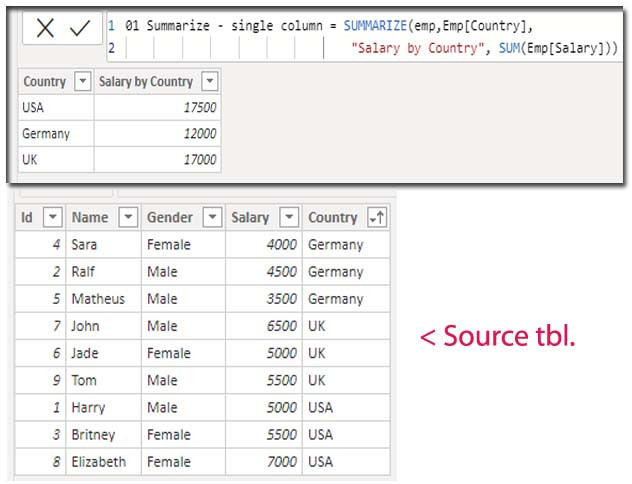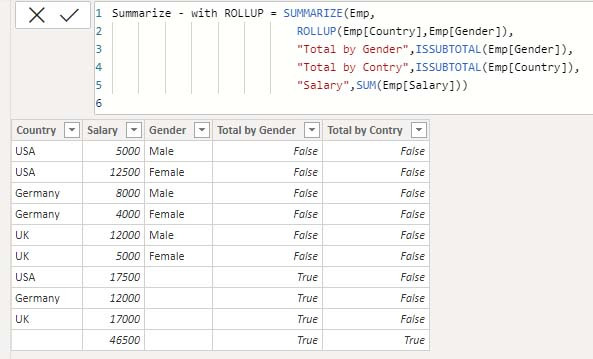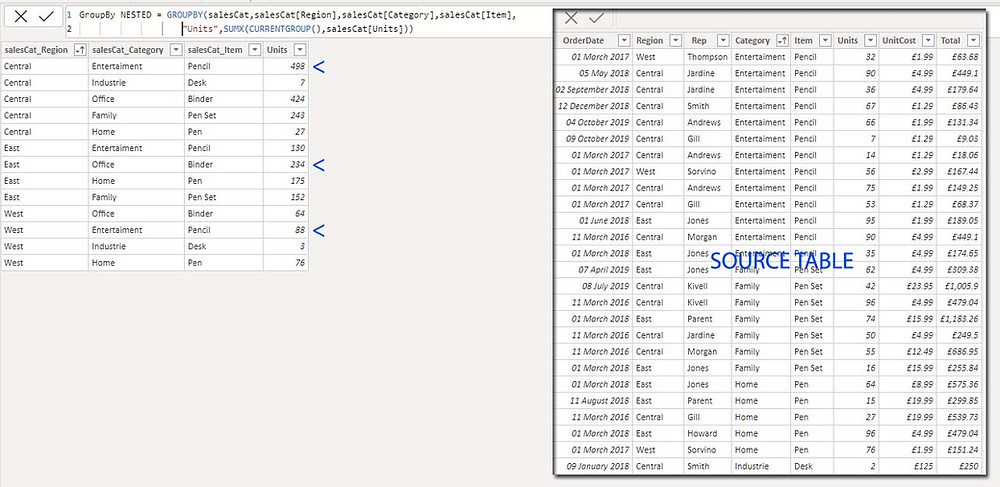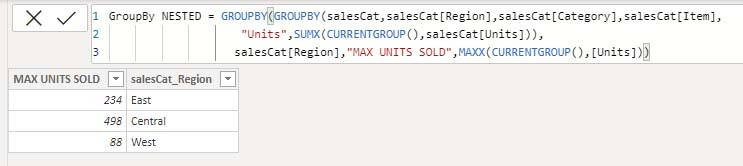Search
• Marek Vavrovic

# SUMMARIZECOLUMNS, SUMMARIZE, GROUPBY

Updated: Sep 13, 2021

03 GROUPBY

01 SUMMARIZECOLUMNS

(Table manipulation functions )

## SUMMARIZECOLUMNS

Returns a summary table.

Use it, if you want to add new table.Remarks

• This function does not guarantee any sort order for the results.

• A column cannot be specified more than once in the groupBy_columnName parameter.

• This function is not supported for use in DirectQuery mode when used in calculated columns or row-level security (RLS) rules.

Example 1

returns a distinct values from a column.Example 2

returns a filtered table.Example 3

returns a filtered table with a subtotal for gender column where country is UKExample 4

using multiple expressions with filtered table.Example 5

With IGNORE

Table is grouped by CustomerID column. If you use IGNORE, a categories with blank values will be return.Example 6

ROLLUPADDISSUBTOTAL returns a grand total per CustomerID. CustomerID can be displayed just ones in the formula, otherwise we got error. Sum function returns a subtotal, together with IGNORE returning the blank values.## SUMMARIZE

Returns a summary table.

Syntax:

SUMMARIZE (<table>, <groupBy_columnName>[, <groupBy_columnName>]…[, <name>, <expression>]…)

### Parameters

table Any DAX expression that returns a table of data.

groupBy_ColumnName(Optional) The qualified name of an existing column used to create summary groups based on the values found in it. This parameter cannot be an expression.

name The name given to a total or summarize column, enclosed in double quotes.

expression Any DAX expression that returns a single scalar value, where the expression is to be evaluated multiple times (for each row/context).

Example 1

returns unique values.Example 2

Emp : table name

Emp[Country]: column name I want to use to summarize (group) the data.

"Salary by Country" : New added column

SUM(Emp[Salary]): type of aggregation for the grouped data.Example 3

with FILTERExample 4

with FILTER

Filters: Continent is Europe, Gender is Female

Summarizing by Country columnExample 5

with ROLLUPExample 6

with ISSUBTOTAL

"Total by Gender" : new column name

ISSUBTOTAL(Emp[Gender]) : function and column name for which I want to return true or false## GROUPBY

The GROUPBY function is similar to the SUMMARIZE function. However, GROUPBY does not do an implicit CALCULATE for any extension columns that it adds.

Example 1

returns a single column, list of the unique values.Example 2

###### With CURRENTGROUP

The CURRENTGROUP function takes no arguments and is only supported as the first argument to one of the following aggregation functions: AVERAGEX, COUNTAX, COUNTX, GEOMEANX, MAXX, MINX, PRODUCTX, STDEVX.S, STDEVX.P, SUMX, VARX.S, VARX.P.

As you can see from this picture below, GROUPBY is not collaborating with all the aggregate functions. It supports only “X” aggregations function with CURRENTGROUP function.working...Example 3

Nested GROUPBY

I want to return these max values by region

3.13.2 Nested GROUPBY

GroupBy NESTED =

GROUPBY (

GROUPBY (

salesCat,

salesCat[Region],

salesCat[Category],

salesCat[Item],

"Units", SUMX ( CURRENTGROUP (), salesCat[Units] )

),

salesCat[Region],

"MAX UNITS SOLD", MAXX ( CURRENTGROUP (), [Units] )

)Example 4

GROUPBY with Filter

GROUPBY with Filter =

FILTER (

GROUPBY (

salesCat,

salesCat[Region],

salesCat[Category],

"Total sales", SUMX ( CURRENTGROUP (), salesCat[Units] * salesCat[UnitCost] )

),

salesCat[Region] = "West"

)Example 5

this combination of code supports CALCULATE function and there's no need to use CURRENTGROUP function.

SYNTAX

PARAMETERS

Term Definition

table Any DAX expression that returns a table of data.

name The name given to the column, enclosed in double quotes.

expression Any DAX expression that returns a scalar expression.

GROUPBY ( Emp, Emp[Country], Emp[Gender] ),

"HEADCOUNT", CALCULATE ( COUNT ( Emp[Name] ) ),

"TOTAL SALARY", CALCULATE ( SUM ( Emp[Salary] ) )

)

Note: must use CALCULATE, otherwise SUM function is not working correctly.Example 6

GROUPBY wth ADDCOLUMNS FILTER = FILTER( ADDCOLUMNS( GROUPBY(salesCat,salesCat[Region],salesCat[Category]), "COUNT UNITS SOLD",CALCULATE(SUM(salesCat[Units]))), salesCat[Region]="West")